Test: Building With Bricks - 1

# Test: Building With Bricks - 1

Test Description

## 10 Questions MCQ Test Mathematics for Class 4: NCERT | Test: Building With Bricks - 1

Test: Building With Bricks - 1 for Class 4 2022 is part of Mathematics for Class 4: NCERT preparation. The Test: Building With Bricks - 1 questions and answers have been prepared according to the Class 4 exam syllabus.The Test: Building With Bricks - 1 MCQs are made for Class 4 2022 Exam. Find important definitions, questions, notes, meanings, examples, exercises, MCQs and online tests for Test: Building With Bricks - 1 below.
Solutions of Test: Building With Bricks - 1 questions in English are available as part of our Mathematics for Class 4: NCERT for Class 4 & Test: Building With Bricks - 1 solutions in Hindi for Mathematics for Class 4: NCERT course. Download more important topics, notes, lectures and mock test series for Class 4 Exam by signing up for free. Attempt Test: Building With Bricks - 1 | 10 questions in 20 minutes | Mock test for Class 4 preparation | Free important questions MCQ to study Mathematics for Class 4: NCERT for Class 4 Exam | Download free PDF with solutions
 1 Crore+ students have signed up on EduRev. Have you?
Test: Building With Bricks - 1 - Question 1

### What is the area of this floor plan?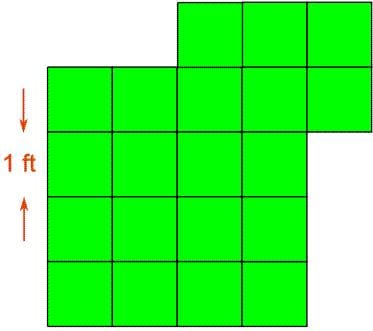Detailed Solution for Test: Building With Bricks - 1 - Question 1

The answer is 20 ft2 because there are altogether 20 squares and each square is 1 ft2

Test: Building With Bricks - 1 - Question 2

### Which statement about the figures is true?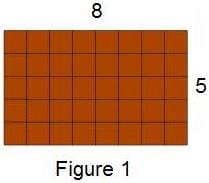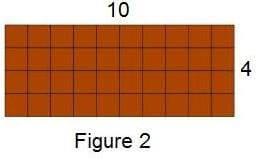Detailed Solution for Test: Building With Bricks - 1 - Question 2

Figure 1's width is 8 and Figure 2's width is 10, so B is incorrect.
Figure 1's height is 5 and Figure 2's height is 4, so C is incorrect.
Figure 1's perimeter is 26 and Figure 2's perimeter is 28, so D is incorrect.
But Figure 1 and Figure 2 both consist of 40 squares, so their areas are equal and A is correct.

Test: Building With Bricks - 1 - Question 3

### What is the area of this shape?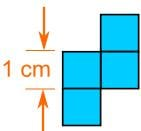Detailed Solution for Test: Building With Bricks - 1 - Question 3

The answer is 4 cm2 because there are 4 squares that are 1 cm2 each

Test: Building With Bricks - 1 - Question 4

The picture below shows the letter U drawn on grid paper. How many squares does the letter U cover?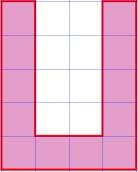Detailed Solution for Test: Building With Bricks - 1 - Question 4

You just have to count them.
There are 12.

Test: Building With Bricks - 1 - Question 5

What is the area of this floor plan?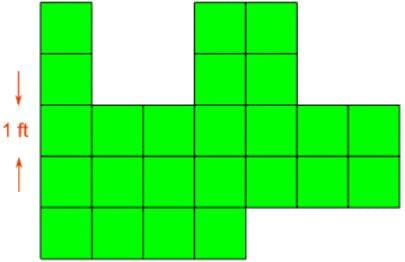Detailed Solution for Test: Building With Bricks - 1 - Question 5

The answer is 24 ft2 because there are 24 squares altogether and each square is 1 ft2

Test: Building With Bricks - 1 - Question 6

What is the area of this shape?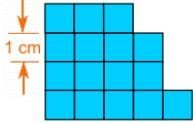Detailed Solution for Test: Building With Bricks - 1 - Question 6

The answer is 16 cm2 because there are 16 squares that are 1 cm2 each

Test: Building With Bricks - 1 - Question 7

What is the area of this picture frame?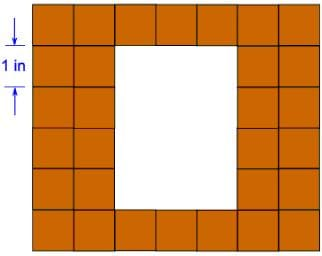Detailed Solution for Test: Building With Bricks - 1 - Question 7

The answer is 30 in.2 because there are altogether 30 squares and each square is 1 in.2

Test: Building With Bricks - 1 - Question 8

What is the area of this shape?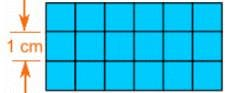Detailed Solution for Test: Building With Bricks - 1 - Question 8

The answer is 18 cm2 because there are
3 × 6 = 18 squares
that are 1 cm2 each

Test: Building With Bricks - 1 - Question 9

What is the area of this shape?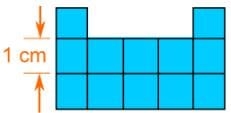Detailed Solution for Test: Building With Bricks - 1 - Question 9

The answer is 12 cm2 because there are 12 squares that are 1 cm2 each

Test: Building With Bricks - 1 - Question 10

John has a chocolate bar consisting of square pieces 1 cm x 1 cm in size. He has already eaten some of the corner pieces (see the picture). How many pieces does John have left?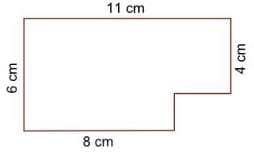Detailed Solution for Test: Building With Bricks - 1 - Question 10

The total number of pieces = 11 × 6 = 66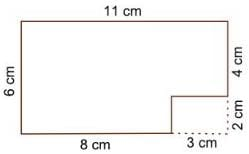The number of pieces he's already eaten = 3 × 2 = 6
Therefore John has 60 pieces left.

## Mathematics for Class 4: NCERT

24 videos|44 docs|32 tests
 Use Code STAYHOME200 and get INR 200 additional OFF Use Coupon Code
Information about Test: Building With Bricks - 1 Page
In this test you can find the Exam questions for Test: Building With Bricks - 1 solved & explained in the simplest way possible. Besides giving Questions and answers for Test: Building With Bricks - 1, EduRev gives you an ample number of Online tests for practice

## Mathematics for Class 4: NCERT

24 videos|44 docs|32 tests